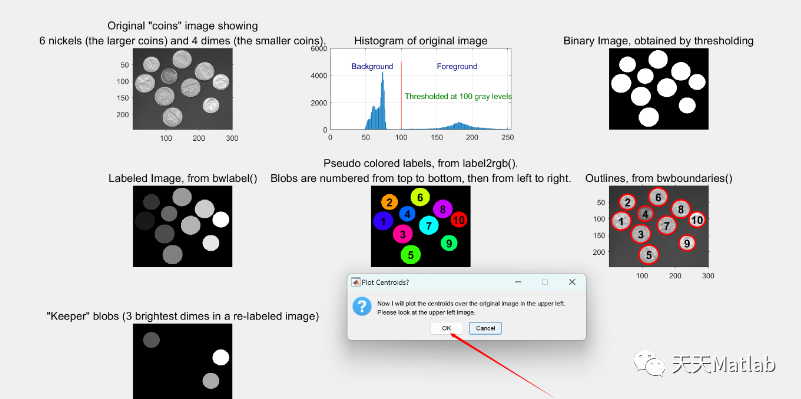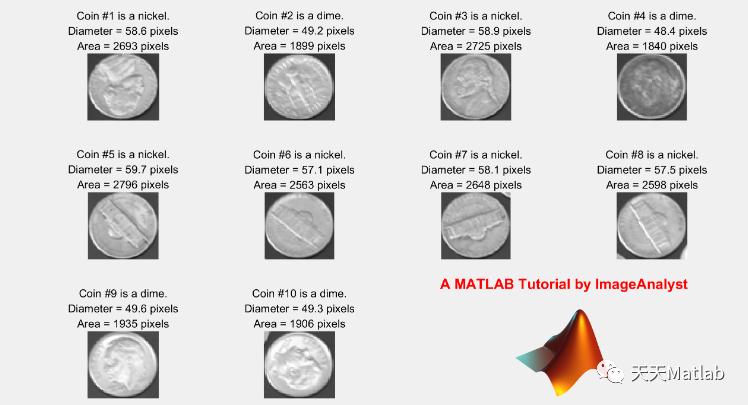# 【图像分割】图像检测（分割、特征提取）、各种特征（面积等）的测量和过滤（Matlab代码实现）

+关注继续查看

✅作者简介：热爱科研的Matlab仿真开发者，修心和技术同步精进，matlab项目合作可私信。

🍎个人主页：Matlab科研工作室

🍊个人信条：格物致知。

## 📣 部分代码

% Grab a cached value if stored.  Here,%% storage - Cache of values and points where these values were generated.%     We need its structure to be something like storage.x{i}, storage.y{i}%     for the points and storage.v{i} for the values.% point - Cell array of structures that contain the location of the cached%     point% value - Name of the value to be returnedfunction [ret storage] = getCached(storage,point,value)    % Check that we have initialized the storage    if ~isstruct(storage)        ret = [];        return;    end    % Check that there's a value cached    if ~isfield(storage,value)        ret = []        return;    end    % Loop over the various cached points    for i = 1:length(storage.(value))        % Loop over the names in the point that we're checking        names = fieldnames(point);        match = true;        for j=1:length(names)            % If the storage doesn't contained the cached point or if the cached            % point differs from queried point, then we're not cached            if  ~isfield(storage,names{j}) || ...                ~isequal(storage.(names{j}){i},point.(names{j}))                match = false;            end            % If we match the point, return the value            if match                ret = storage.(value){i};                break;            % Otherwise, return something empty            else                ret = [];            end        end        % If we've successfully cached a value, break out        if ~isempty(ret)            break;        end    end    % If we found the cached value, reshuffle things so this point and value is    % first in the cached storage    if ~isempty(ret)        % Loop over the names in the point that we're checking        names = fieldnames(point);        for j=1:length(names)            start = storage.(names{j})(i);            middle = storage.(names{j})(1:i-1);            last = storage.(names{j})(i+1:end);            storage.(names{j}) = [start,middle,last];        end    endend

## ⛳️ 运行结果## 🔗 参考文献

 陈海永,孙鹤旭,徐德.一类窄焊缝的结构光图像特征提取方法[J].焊接学报, 2012(001):033.

 罗帅.基于X射线的焊缝缺陷图像特征提取研究[D].电子科技大学,2016.

### 👇  私信完整代码和数据获取及论文数模仿真定制

#### 9 雷达方面

##### 卡尔曼滤波跟踪、航迹关联、航迹融合

|
5天前
Matlab随机变量的数字特征（一）
Matlab随机变量的数字特征
23 0
|
5天前
|
Perl
Matlab随机变量的数字特征（二）
Matlab随机变量的数字特征
18 0
|
2月前
|

Elman分类预测 | Matlab基于递归神经网络Elman分类预测，多特征输入模型
Elman分类预测 | Matlab基于递归神经网络Elman分类预测，多特征输入模型
42 1
|
3月前
|

Matlab 基于PSO-GRU-Attention做多特征输入单输出的预测模型
Matlab 基于PSO-GRU-Attention做多特征输入单输出的预测模型
40 0
|
3月前
|

Matlab 基于PSO-LSTM-Attention做多特征输入单输出的预测模型
Matlab 基于PSO-LSTM-Attention做多特征输入单输出的预测模型
33 0
|
3月前
|

53 3
|
4月前
|

40 0
|
4月前
|

46 0
|
4月前
|

【使用深度学习的城市声音分类】使用从提取音频特征（频谱图）中提取的深度学习进行声音分类研究（Matlab代码实现）
【使用深度学习的城市声音分类】使用从提取音频特征（频谱图）中提取的深度学习进行声音分类研究（Matlab代码实现）
55 0
|
4月前
|

29 0